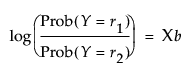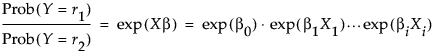Publication date: 03/23/2021

## Odds Ratios

For two response levels, the logistic regression model is specified as follows:where r1 and r1 are the two response levels

Therefore, the odds are defined as follows:Note that exp(βi(Xi + 1)) = exp(βiXi) exp(βi). This shows that if Xi changes by a unit amount, the odds is multiplied by exp(βi), which we label the unit odds ratio. As Xi changes over its whole range, the odds are multiplied by exp((Xhigh - Xlow)βi), which we label the range odds ratio. For binary responses, the log odds ratio for flipped response levels involves only changing the sign of the parameter. Therefore, you might want the reciprocal of the reported value to focus on the last response level instead of the first.

Two-level nominal effects are coded 1 and -1 for the first and second levels, so range odds ratios or their reciprocals would be of interest.

If no confidence intervals for the parameter estimates have been calculated when you select the Odds Ratio option, selecting the Odds Ratio option produces Wald-based confidence intervals for the odds ratio.

Selecting the Odds Ratio option produces profile likelihood-based confidence intervals for the odds ratios when all of the following conditions are met:

the Likelihood Ratio Tests option has been selected

the Confidence Intervals option has been selected

the number of parameters for each response is less than 8

the number of rows is less than 1000.

The method used for computing confidence intervals for the odds ratios is noted at the bottom of the Odds Ratios report.

Want more information? Have questions? Get answers in the JMP User Community (community.jmp.com).